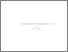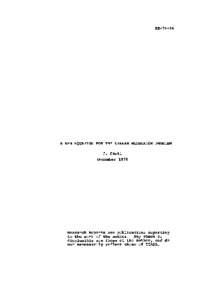# A New Equation for the Linear Regulator Problem

Casti, J.L. (1974). A New Equation for the Linear Regulator Problem. IIASA Research Report. IIASA, Laxenburg, Austria: RR-74-024Preview
Text
RR-74-024.pdf

## Abstract

In this article a new equation is derived for the optimal feedback gain matrix characterizing the solution of the standard linear regulator problem. It will be seen that, in contrast to the usual algebraic Riccati equation which requires the solution of n (n+1)/2 quadratically nonlinear algebraic equations, the new equation requires the solution of only nm such equations, where m is the number of system input terminals, and n is the dimension of the state vector of the system.

Utilizing the new equation, results are presented for the inverse problem of linear control theory.

Item Type: Monograph (IIASA Research Report) System and Decision Sciences - Core (SDS) IIASA Import 15 Jan 2016 01:40 27 Aug 2021 17:07 http://pure.iiasa.ac.at/70View Item